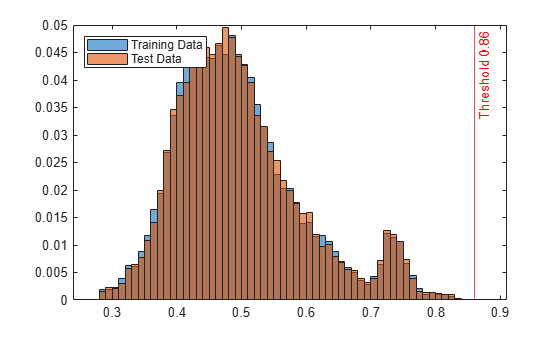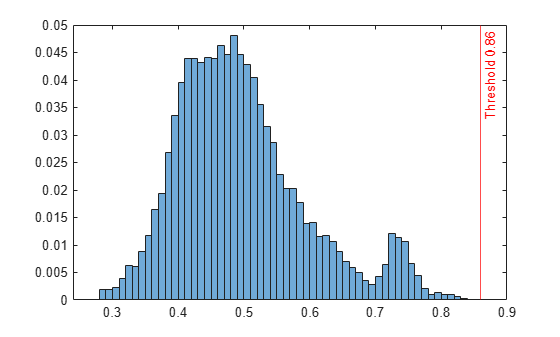# isanomaly

Find anomalies in data using isolation forest

## Syntax

``tf = isanomaly(forest,Tbl)``
``tf = isanomaly(forest,X)``
``tf = isanomaly(___,Name=Value)``
``[tf,scores] = isanomaly(___)``

## Description

example

````tf = isanomaly(forest,Tbl)` finds anomalies in the table `Tbl` using the `IsolationForest` object `forest` and returns the logical array `tf`, whose elements are `true` when an anomaly is detected in the corresponding row of `Tbl`. You must use this syntax if you create `forest` by passing a table to the `iforest` function.```
````tf = isanomaly(forest,X)` finds anomalies in the matrix `X`. You must use this syntax if you create `forest` by passing a matrix to the `iforest` function.```

example

````tf = isanomaly(___,Name=Value)` specifies options using one or more name-value argument combinations in addition to any of the input arguments in the previous syntaxes. For example, set `ScoreThreshold=0.5` to identify observations with scores above 0.5 as anomalies.```
````[tf,scores] = isanomaly(___)` also returns an anomaly score in the range `[0,1]` for each observation in `Tbl` or `X`. A score value close to 0 indicates a normal observation, and a value close to 1 indicates an anomaly.```

## Examples

collapse all

Create an `IsolationForest` object for uncontaminated training observations by using the `iforest` function. Then detect novelties (anomalies in new data) by passing the object and the new data to the object function `isanomaly`.

Load the 1994 census data stored in `census1994.mat`. The data set consists of demographic data from the US Census Bureau to predict whether an individual makes over \$50,000 per year.

`load census1994`

`census1994` contains the training data set `adultdata` and the test data set `adulttest`.

Train an isolation forest model for `adultdata`. Assume that `adultdata` does not contain outliers.

```rng("default") % For reproducibility [Mdl,tf,s] = iforest(adultdata);```

`Mdl` is an `IsolationForest` object. `iforest` also returns the anomaly indicators `tf` and anomaly scores `s` for the training data `adultdata`. If you do not specify the `ContaminationFraction` name-value argument as a value greater than 0, then `iforest` treats all training observations as normal observations, meaning the values in `tf` are all logical 0 (`false`). The function sets the score threshold to the maximum score value. Display the threshold value.

`Mdl.ScoreThreshold`
```ans = 0.8600 ```

Find anomalies in `adulttest` by using the trained isolation forest model.

`[tf_test,s_test] = isanomaly(Mdl,adulttest);`

The `isanomaly` function returns the anomaly indicators `tf_test` and scores `s_test` for `adulttest`. By default, `isanomaly` identifies observations with scores above the threshold (`Mdl.ScoreThreshold`) as anomalies.

Create histograms for the anomaly scores `s` and `s_test`. Create a vertical line at the threshold of the anomaly scores.

```histogram(s,Normalization="probability") hold on histogram(s_test,Normalization="probability") xline(Mdl.ScoreThreshold,"r-",join(["Threshold" Mdl.ScoreThreshold])) legend("Training Data","Test Data",Location="northwest") hold off```Display the observation index of the anomalies in the test data.

`find(tf_test)`
```ans = 15655 ```

The anomaly score distribution of the test data is similar to that of the training data, so `isanomaly` detects a small number of anomalies in the test data with the default threshold value. You can specify a different threshold value by using the `ScoreThreshold` name-value argument. For an example, see Specify Anomaly Score Threshold.

Specify the threshold value on anomaly scores by using the `ScoreThreshold` name-value argument of `isanomaly`.

Load the 1994 census data stored in `census1994.mat`. The data set consists of demographic data from the US Census Bureau to predict whether an individual makes over \$50,000 per year.

`load census1994`

`census1994` contains the training data set `adultdata` and the test data set `adulttest`.

Train an isolation forest model for `adultdata`.

```rng("default") % For reproducibility [Mdl,tf,scores] = iforest(adultdata);```

Plot a histogram of the score values. Create a vertical line at the default score threshold.

```histogram(scores,Normalization="probability"); xline(Mdl.ScoreThreshold,"r-",join(["Threshold" Mdl.ScoreThreshold]))```Find the anomalies in the test data using the trained isolation forest model. Use a different threshold from the default threshold value obtained when training the isolation forest model.

Determine the score threshold by using the `isoutlier` function.

`[~,~,U] = isoutlier(scores)`
```U = 0.7449 ```

Specify the `ScoreThreshold` name-value argument value as `U`.

```[tf_test,scores_test] = isanomaly(Mdl,adulttest,ScoreThreshold=U); histogram(scores_test,Normalization="probability") xline(U,"r-",join(["Threshold" U]))```## Input Arguments

collapse all

Trained isolation forest model, specified as an `IsolationForest` object.

Predictor data, specified as a table. Each row of `Tbl` corresponds to one observation, and each column corresponds to one predictor variable. Multicolumn variables and cell arrays other than cell arrays of character vectors are not allowed.

If you train `forest` using a table, then you must provide predictor data by using `Tbl`, not `X`. All predictor variables in `Tbl` must have the same variable names and data types as those in the training data. However, the column order in `Tbl` does not need to correspond to the column order of the training data.

Data Types: `table`

Predictor data, specified as a numeric matrix. Each row of `X` corresponds to one observation, and each column corresponds to one predictor variable.

If you train `forest` using a matrix, then you must provide predictor data by using `X`, not `Tbl`. The variables that make up the columns of `X` must have the same order as the training data.

Data Types: `single` | `double`

### Name-Value Arguments

Specify optional pairs of arguments as `Name1=Value1,...,NameN=ValueN`, where `Name` is the argument name and `Value` is the corresponding value. Name-value arguments must appear after other arguments, but the order of the pairs does not matter.

Example: `ScoreThreshold=0.75,UseParallel=true` sets the threshold for the anomaly score to 0.75 and instructs the function to run computations in parallel.

Threshold for the anomaly score, specified as a numeric scalar between 0 and 1. `isanomaly` identifies observations with scores above the threshold as anomalies.

The default value is the `ScoreThreshold` property value of `forest`.

Example: `ScoreThreshold=0.5`

Data Types: `single` | `double`

Flag to run in parallel, specified as `true` or `false`. If you specify `UseParallel=true`, the `isanomaly` function executes for-loop iterations in parallel by using `parfor`. This option requires Parallel Computing Toolbox™.

Example: `UseParallel=true`

Data Types: `logical`

## Output Arguments

collapse all

Anomaly indicators, returned as a logical column vector. An element of `tf` is `true` when the observation in the corresponding row of `Tbl` or `X` is an anomaly, and `false` otherwise. `tf` has the same length as `Tbl` or `X`.

`isanomaly` identifies observations with `scores` above the threshold (the `ScoreThreshold` value) as anomalies.

Anomaly scores, returned as a numeric column vector whose values are between 0 and 1. `scores` has the same length as `Tbl` or `X`, and each element of `scores` contains an anomaly score for the observation in the corresponding row of `Tbl` or `X`. A score value close to 0 indicates a normal observation, and a value close to 1 indicates an anomaly.

collapse all

### Isolation Forest

The isolation forest algorithm  detects anomalies by isolating anomalies from normal points using an ensemble of isolation trees.

The `iforest` function builds an isolation forest (ensemble of isolation trees) for training observations and detects outliers (anomalies in the training data). Each isolation tree is trained for a subset of training observations, sampled without replacements. `iforest` grows an isolation tree by choosing a split variable and split position at random until every observation in a subset lands in a separate leaf node. Anomalies are few and different; therefore, an anomaly lands in a separate leaf node closer to the root node and has a shorter path length (the distance from the root node to the leaf node) than normal points. The function identifies outliers using anomaly scores defined based on the average path lengths over all isolation trees.

The `isanomaly` function uses a trained isolation forest to detect anomalies in data. For novelty detection (detecting anomalies in new data with uncontaminated training data), you can train an isolation forest with uncontaminated training data (data with no outliers) and use it to detect anomalies in new data. For each observation of the new data, the function finds the average path length to reach a leaf node from the root node in the trained isolation forest, and returns an anomaly identifier and score.

For more details, see Anomaly Detection with Isolation Forest.

### Anomaly Scores

The isolation forest algorithm computes the anomaly score s(x) of an observation x by normalizing the path length h(x):

`$s\left(x\right)={2}^{-\frac{E\left[h\left(x\right)\right]}{c\left(n\right)}},$`

where E[h(x)] is the average path length over all isolation trees in the isolation forest, and c(n) is the average path length of unsuccessful searches in a binary search tree of n observations.

• The score approaches 1 as E[h(x)] approaches 0. Therefore, a score value close to 1 indicates an anomaly.

• The score approaches 0 as E[h(x)] approaches n – 1. Also, the score approaches 0.5 when E[h(x)] approaches c(n). Therefore, a score value smaller than 0.5 and close to 0 indicates a normal point.

## Algorithms

`isanomaly` considers `NaN`, `''` (empty character vector), `""` (empty string), `<missing>`, and `<undefined>` values in `Tbl` and `NaN` values in `X` to be missing values.

• `isanomaly` does not use observations with all missing values and assigns the anomaly score of 1 to the observations.

• `isanomaly` uses observations with some missing values to find splits on variables for which these observations have valid values.

 Liu, F. T., K. M. Ting, and Z. Zhou. "Isolation Forest," 2008 Eighth IEEE International Conference on Data Mining. Pisa, Italy, 2008, pp. 413-422.